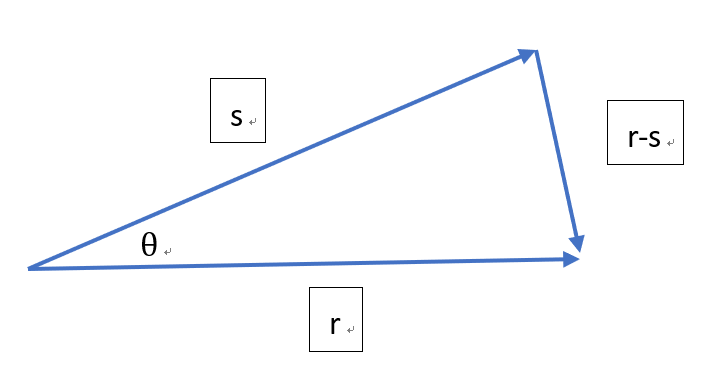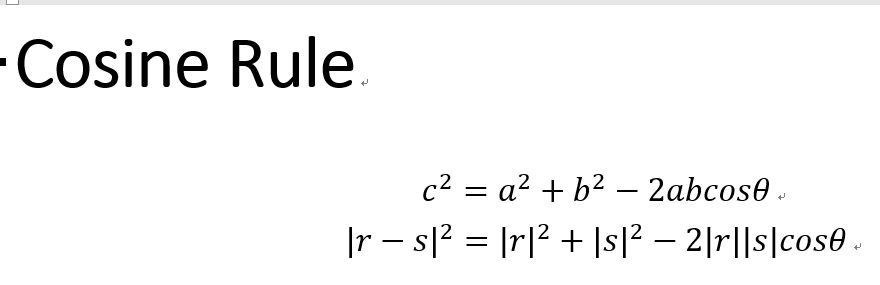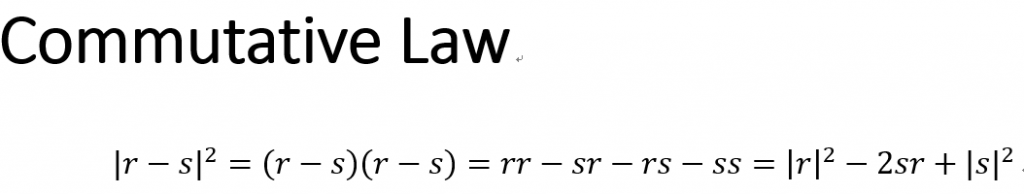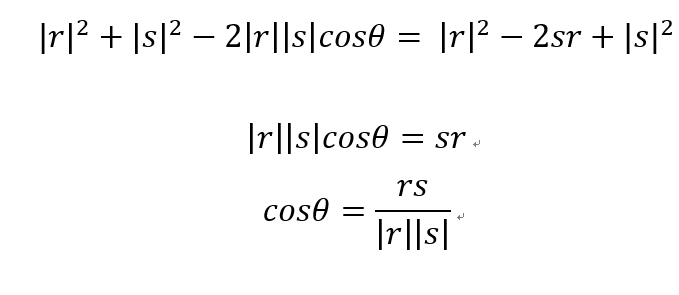#2019 iT 邦幫忙鐵人賽DAY 12
0

## Day 12-機器學習與數學天天玩-PCA-Transformation of Vectors in Spaces: Dot Product Part 2We are now in the PCA journey, station 2.

1. Statistical Introduction
2. Transformation of Vectors in Spaces
2. Orthogonal Projection

To solve the problem of calculating angles between two vectors, we have to use 2 concepts, consine rule and commutative law.Becasue consine rule and commutative law work as follows:We can get the angle of cosine: